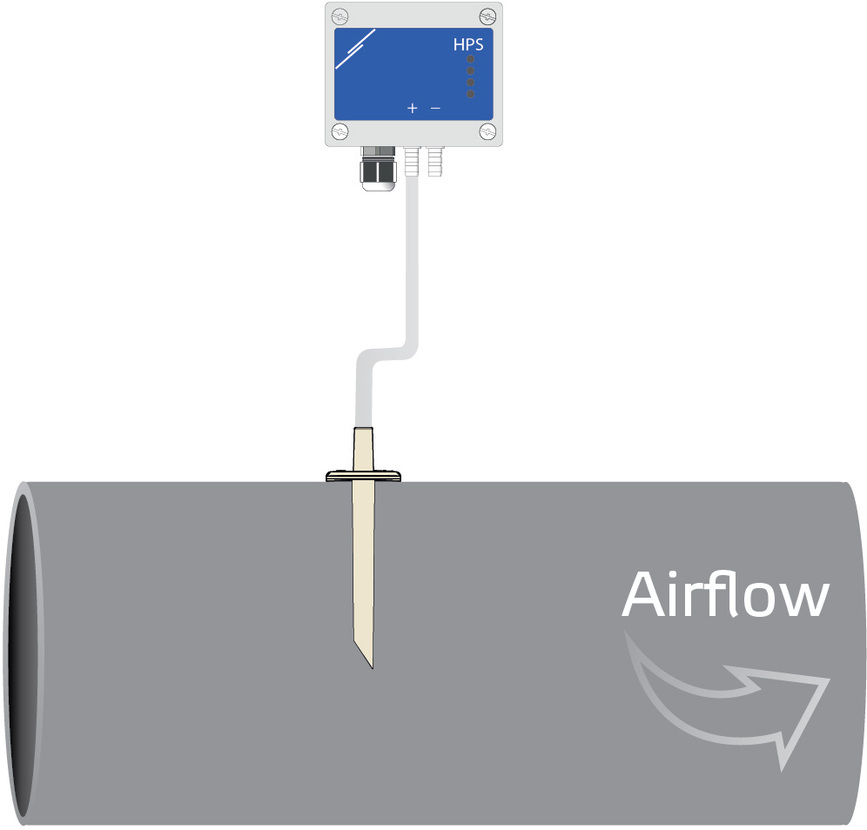Sentera – your partner in HVAC control solutions
Category
• HVAC control solutions
• Customer service
• Senteraweb - Setup, monitoring, logging

# How to measure air volume flow or air velocity?

A balanced ventilation system supplies fresh air to the building while it extracts stale air from the building. To check whether there is sufficient ventilation in a duct, the supply of fresh air must be measured and regulated. The amount of fresh air supplied into the building or the air volume flow of fresh air must therefore be measured. Air volume flow is usually measured in m³ per hour (m³/h).

Parameters
In order to measure airflow or the volume flow, there are a few important parameters to know:

- Duct cross-section: the area between the edges of the duct, squared. This is denoted in mm squared (mm²).
- Differential pressure: The difference between the pressure outside the duct (static pressure) and inside the duct (dynamic pressure). (Differential) pressure is denoted in Pascal (Pa).
- Air velocity: the speed at which the air flows. It is denoted in meters per second (m/s)
- K-factor: a representation of the combination of other parameters that can impact the air velocity and airflow inside the Pitot tube. This is a set number provided by the manufacturer. It mostly corrects for the disturbances in laminar flow caused by the exhaust. This is a number without denotation.
- Air density: the density of the air flowing through the duct. This should be about 1,2 kg/m³ in normal indoor environments. It can slightly change depending on the ambient conditions.

Formulas
These parameters are all interconnected in the environment of a ventilation duct. The formulas we use to calculate the parameters are based on Bernoulli’s Principle. This principle states that an increase in the speed of a fluid or gas occurs at the same time as a decrease in the potential energy. To calculate air velocity and airflow, the following formulas are used:

Air velocity is √ (2 * Differential pressure/air density)

Airflow is duct cross-section * √ (2 * Differential pressure/air density)

Sensors and accessories
Airflow or volume flow can be measured with the Sentera HPS or DPS series of differential pressure sensors.  Regulating airflow requires a differential pressure controller. These controllers have article codes HPSP, HPSA, DPSP, DPSA, and SPS2

The following accessories can be used to connect the sensor to the air duct where the airflow is to be measured:

PSET-PVC-200 can be used to measure differential pressure [Pa]. If the K-factor is known, it can also be used to control air velocity.
The formula for air velocity is √ (2 * Differential pressure/air density * K-factor).

For example:
The sensor measures 25 Pa differential pressure. The K-factor is 1,04. The air velocity would be √ (2 * 25 Pa/1,2 kg/m³ * 1,04) = 6,58 meters / second.

Or:

PSET-PTS-200 can be used to measure air velocity [m/s]. If the duct cross-section is known, it can be used to control air volume flow. By multiplying air velocity by the cross-section area of a duct, the sensor calculates the air volume flowing past a point in the duct per unit of time.

The formula for air volume flow is therefore air velocity * cross sectional area of the duct OR √ (2 * Differential pressure/air density * K-factor) * cross sectional area of the duct.

If we take the previous example, and take a standard duct size of 125 mm, we would get the following equation:
6,58 m/s * 0,0122 m² = 0,08 m³/s, or 289 m³/h.Website is not supported on Internet Explorer! Please use Chrome, Firefox, Edge or other browser.